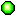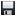Tracking budget swap between projects requires you to create several new attributes. These attributes are used to identify the source of approved budget dollars in a project, as well as calculate total budget dollars and total budget transfers between projects.

## Attribute List

The following list describes the attributes you need to create for this scenario, the uses for each, and the location that each appears in the interface:
• cs Budget Transfer Effective Date This attribute is used to determine when the budget swap took place. It appears on the Dependency Creation dialog
• cs Percentage Transfer This attribute appears on the Dependency Creation dialog. The value entered is used by the Percentage Budget attribute in calculating the total dollar value of the budget swap
• cs Self Budget This attribute appears on the Summary view for the Project item type. The dollar value entered for this attribute represents the original approved budget for the Project
• cs Total Budget This attribute appears on the Summary view for the Project item type and contains the calculated total dollar value for the project budget
• cs Constant End Date This attribute is used in calculating the input and output budget values
• cs Percentage Budget This attribute appears on the Dependency Creation dialog and calculates the total dollar value of the budget swap
• cs Range Input Budget This attribute appears on the Summary view for the Project item type. It calculates the total value of budget dollars reallocated to the selected project from other projects
• cs Range Output Budget This attribute appears on the Summary view for the Project item type. It calculates the total value of budget dollars swapped out of the selected project and reallocated to other projects
• cs Timephased Input Budget This is a calculated attribute the lives on the dependency. It is used to roll up the reallocated budget dollars to the target project
• cs Timephased Output Budget This is a calculated attribute the lives on the dependency. It is used to roll up the reallocated budget dollars to the source project

The following table provides the properties for each attribute described above. In creating attributes, you enter the attribute name and default label. The system generates an XML name for each attribute, which is used in MathML calculations. The XML name value is not editable, but is provided in the table below as reference.

Table 1.
Attribute Name XML Name Type Rollup Type Calculated
cs Budget Transfer Effective Date cs-Budget-Transfer-Effective-Date Date None No
cs Percentage Transfer cs-Percentage-Transfer Percent None No
cs Self Budget cs-Self-Budget Money Sum No
cs Total Budget cs-Total-Budget Money None No
cs Constant End Date cs-Constant-End-Date Date None No
cs Percentage Budget cs-Percentage-Budget Money None Yes
cs Range Input Budget cs-Range-Input-Budget Ranged Calculation None Yes
cs Range Output Budget cs-Range-Output-Budget Ranged Calculation None Yes
cs Timephased Input Budget cs-Timephased-Input-Budget Timephased Currency Sum Yes
cs Timephased Output Budget cs-Timephased-Output-Budget Timephased Currency Sum Yes

Use the following procedure to create the custom date attributes (cs Budget Transfer Effective Date and cs Constant End Date).

2. Select Customization.
3. Select the Attributes Setup tab.
4. Select the Attributes tab.
5. Click New.
6. Name the new attribute. The system name will be generated automatically.
7. From the Type drop-down, select date.
8. From the Rollup Type drop-down, select none (default, no roll-up), min (selects the lowest value or earliest date from an attribute's direct descendent values), max (selects the highest value or latest date from an attribute's direct descendent values), sum (to add all of this attribute's direct descendent values). General attributes that use the sum roll-up type do not require a dependency. An attribute with a rollup type other than none will be saved as a global attribute.
9. Select Global to make the attribute a global attribute. A global attribute is required for all item types and optional for all work types.
10. Select Searchable to allow users to search for the attribute in the Projects and Reports modules.
11. Under List Attributes, choose an attribute list to which the attribute will be associated.
12. Under Calculation, select Calculated and then click Calculation to open the Calculation Editor dialog box.
13. Calculated attributes can be associated with dimensions (except when using Serena Agile On Demand). Under Dimensions, select Dimensioned and then choose the dimensions to which the attribute is associated. If you want to use a MathML calculation with the calculated timephased attribute, select Calculated and then click Calculation to open the Calculation Editor dialog box. Add the MathML calculation and click OK.
14. Click Save.

Use the following procedure to create the custom percent attributes (cs Percentage Transfer).

2. Select Customization.
3. Select the Attributes Setup tab.
4. Select the Attributes tab.
5. Click New.
6. Name the new attribute. The system name will be generated automatically.
7. From the Type drop-down, select percent.
8. From the Rollup Type drop-down, select none (default, no roll-up), min (selects the lowest value or earliest date from an attribute's direct descendent values), max (selects the highest value or latest date from an attribute's direct descendent values), sum (to add all of this attribute's direct descendent values). General attributes that use the sum roll-up type do not require a dependency. An attribute with a rollup type other than none will be saved as a global attribute.
9. Select Global to make the attribute a global attribute. A global attribute is required for all item types and optional for all work types.
10. Select Searchable to allow users to search for the attribute in the Projects and Reports modules.
11. Under List Attributes, choose an attribute list to which the attribute will be associated.
12. Under Calculation, select Calculated and then click Calculation to open the Calculation Editor dialog box.
13. Calculated attributes can be associated with dimensions (except when using Serena Agile On Demand). Under Dimensions, select Dimensioned and then choose the dimensions to which the attribute is associated. If you want to use a MathML calculation with the calculated timephased attribute, select Calculated and then click Calculation to open the Calculation Editor dialog box. Add the MathML calculation and click OK.
14. Click Save.

Use the following procedure to create the custom money attributes (cs Self Budget, cs Total Budget, and cs Percentage Budget).

Use the following calculation for cs Percentage Budget:

```          <?xml version="1.0" encoding="utf-16"?>
<Calculation>
<Expression>
<math xmlns:mathml="http://www.w3.org/1998/Math/MathML">
<lambda>
<apply>
<times></times>
<ci>a</ci>
<ci>b</ci>
</apply>
</lambda>
[/itex]
</Expression>
<RHSVariable name="cs-Self-Budget,DepSide=source" identifier="a"></RHSVariable>
<RHSVariable name="cs-Percentage-Transfer" identifier="b"></RHSVariable>
</Calculation>
```

2. Select Customization.
3. Select the Attributes Setup tab.
4. Select the Attributes tab.
5. Click New.
6. Name the new attribute. The system name will be generated automatically.
7. From the Type drop-down, select money.
8. From the Rollup Type drop-down, select none (default, no roll-up), min (selects the lowest value or earliest date from an attribute's direct descendent values), max (selects the highest value or latest date from an attribute's direct descendent values), sum (to add all of this attribute's direct descendent values). General attributes that use the sum roll-up type do not require a dependency. An attribute with a rollup type other than none will be saved as a global attribute.
9. Select Global to make the attribute a global attribute. A global attribute is required for all item types and optional for all work types.
10. Select Searchable to allow users to search for the attribute in the Projects and Reports modules.
11. Under List Attributes, choose an attribute list to which the attribute will be associated.
12. Under Calculation, select Calculated and then click Calculation to open the Calculation Editor dialog box.
13. Calculated attributes can be associated with dimensions (except when using Serena Agile On Demand). Under Dimensions, select Dimensioned and then choose the dimensions to which the attribute is associated. If you want to use a MathML calculation with the calculated timephased attribute, select Calculated and then click Calculation to open the Calculation Editor dialog box. Add the MathML calculation and click OK.
14. Click Save.

Use the following procedure to create the custom ranged calculation attributes (cs Range Input Budget and cs Range Output Budget).

Use the following calculation for cs Range Input Budget:

```          <?xml version="1.0" encoding="utf-16"?>
<Calculation>
<Expression>
<math xmlns:mathml="http://www.w3.org/1998/Math/MathML">
<lambda>
<bvar>
<ci>a</ci>
</bvar>
<bvar>
<ci>b</ci>
</bvar>
<apply>
<plus/>
<ci>a</ci>
</apply>
</lambda>
[/itex]
</Expression>
<RHSVariable name="%RangeCalculation%Sum%cs-Timephased-Input-Budget%Currency%Calendar Month%START%END%" identifier="a"/>
</Calculation>
```

Use the following calculation for cs Range Output Budget:

```          <?xml version="1.0" encoding="utf-16"?>
>Calculation>
>Expression>
>math xmlns:mathml="http://www.w3.org/1998/Math/MathML">
>lambda>
>bvar>
>ci>a>/ci>
>/bvar>
>bvar>
>ci>b>/ci>
>/bvar>
>apply>
>plus/>
>ci>a>/ci>
>/apply>
>/lambda>
>/math>
>/Expression>
>RHSVariable name="%RangeCalculation%Sum%cs-Timephased-Output-Budget%Currency%Calendar Month%START%END%" identifier="a"/>
>/Calculation>
```

To add a ranged calculation attribute

2. Select Customization.
3. Select the Attributes Setup tab.
4. Select the Attributes tab.
5. Click New.
6. Name the new attribute. The system name will be generated automatically.
7. From the Type drop-down, select ranged calculation.
8. From the Rollup Type drop-down, select none (default, no roll-up) or sum (to add all of this attribute's direct descendent values). Ranged calculation attributes that use the sum roll-up type should only be used with a dependency. An attribute with a rollup type other than none will be saved as a global attribute.
9. From the Display Value drop-down, select float, integer, money, or percent.
10. Select Global to make the attribute a global attribute. A global attribute is required for all item types and optional for all work types.
11. Select Searchable to allow users in your organization to find this attribute using search.
12. A calculated timephased attribute can be associated with dimensions. Under Dimensions, select Dimensioned and then choose the dimensions to which the attribute is associated. If you want to use a MathML calculation with the calculated timephased attribute, select Calculated and then click Calculation to open the Calculation Editor dialog box. Add the MathML calculation and click OK.
13. Click Save.

Use the following procedure to create the custom timephased currency attributes (cs Timephased Input Budget and cs Timephased Output Budget).

Use the following calculation for cs Timephased Input Budget:

```          <?xml version="1.0" encoding="utf-16"?>
<Calculation>
<Expression>
<math xmlns:mathml="http://www.w3.org/1998/Math/MathML">
<lambda>
<apply>
<plus></plus>
<ci>a</ci>
</apply>
</lambda>
[/itex]
</Expression>
<RHSVariable name="%TimePhaseScaler%cs-Percentage-Budget%cs-Budget-Transfer-Effective-Date%cs-Constant-End-Date" identifier="a"></RHSVariable>
</Calculation>
```

Use the following calculation for cs Timephased Output Budget:

```          <?xml version="1.0" encoding="utf-16"?>
<Calculation>
<Expression>
<math xmlns:mathml="http://www.w3.org/1998/Math/MathML">
<lambda>
<apply>
<plus></plus>
<ci>a</ci>
</apply>
</lambda>
[/itex]
</Expression>
<RHSVariable name="%TimePhaseScaler%cs-Percentage-Budget%cs-Budget-Transfer-Effective-Date%cs-Constant-End-Date" identifier="a"></RHSVariable>
</Calculation>
```

To add a timephased currency attribute

5. Click New.
8. Click Save.